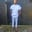Related Tags

go
sorting
communitycreator

# How to do a custom sort in GoChinweuba Elijah Azubuike

### Overview

Sorting is the process of arranging data in a systematic way.

When we want to sort items according to criteria that are different from the normal — for example, if we want to sort strings according to their length rather than their alphabetical order — we can use the custom sorting method.

### Packages

We use the sort and the fmt package to implement this.

### fmt package

The fmt package is used to output the results to the screen, as we will see in the example below.

### sort package

The sort package is used in Go to sort the slices of different data types.

The sort package comes with three interfaces, as explained in the example below.

1. Len()
2. Swap()
3. Less()

In the following example, we sort strings according to their lengths.

### Example

package main

import (
"fmt"
"sort"
)

type byLength []string

func (s byLength) Len() int {
return len(s)
}
func (s byLength) Swap(i, j int) {
s[i], s[j] = s[j], s[i]
}
func (s byLength) Less(i, j int) bool {
return len(s[i]) < len(s[j])// getting the lenght of strings
}

func main() {
fruits := []string{"peach", "banana", "kiwi"}
sort.Sort(byLength(fruits))//calling the sort
fmt.Println(fruits)// outputing results
}

### Interface explanation

• The Less interface: If the element with index i must sort before the one with index j, then Less reports it. The items at index i and j are regarded equal if both Less(i, j) and Less(j, j) are false.

• The Len interface: The number of elements in the collection is denoted by Len.

• The Swap interface: This performs the swapping between the two indices.

### Code explanation

From the example above:

• In lines 3-6, we first import our packages, i.e., the fmt and sort packages.

• In line 8, we declare a byLength type which is an alias of the builtin []string type.

• In line 10-12, we create a function that returns the length of a string.

• In line 16, we use Less and our bylength type to write the logic to get the string with the highest length. We then execute the sorting using our sort package to carry out the custom sorting.

• In lines 21-22, we carry out a sort on the fruit variable where we implement the function we’ve previously created.

• In line 24, we output the result using the fmt package.

RELATED TAGS

go
sorting
communitycreator

CONTRIBUTORChinweuba Elijah Azubuike
RELATED COURSES

View all Courses

Keep Exploring

Learn in-demand tech skills in half the time